TWiki >Manufacturing Web>ManufacturingHome > MaterialsInfo > UrethaneProperties>YoungsModuliForUrethanes (31 Jul 2010, DanAukes)
At Will's suggestion, I have computed the Young's modulus for the 20A polyurethane. If you do similar calculations in the future please put your results here. -- AlanAsbeck - 27 Apr 2005

## Summary of Material properties:

Material Young's Modulus Max. Tensile strength
IE-20A 217 kPa ~190 psi
IE-35A 350 kPa ~230 psi
Very old IE-50A 200kPa ?
IE-60A 563 kPa ~200 psi
Vytaflex 20A 195 kPa ?
Vytaflex 40A 400 kpa ?
Vytaflex 60A 1.2-1.3 MPa ?

## IE-20A:

```Tested: a 20A flexure 7.76 x 0.78 x 1.49 mm.
in F= k*x, k=32.46 N/m using the data between the 20g and 30g points (see figure below).
l = .00776 m
A = 1.1622e-6 m^2
E = k*l/A = 2.17e5 Pa = 217 kPa

you can use the formula
k=E*A/l
to quickly compute how other flexure geometries will behave.
```

• 20A flexure used in testing: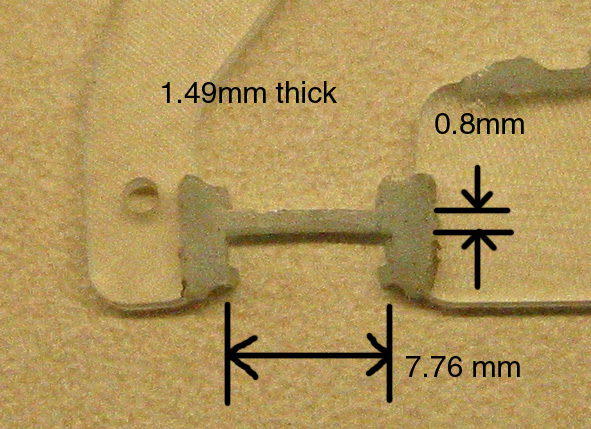• 20Astressstrain2.jpg: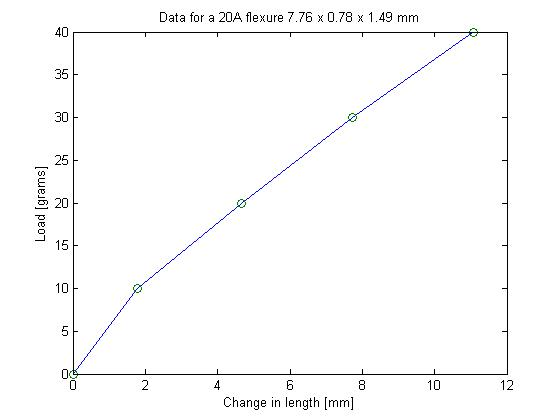Actual data:

Length [mm] Change in length [mm] Load [g]
7.76 0 0
9.55 1.79 10
12.41 4.65 20
15.49 7.73 30
18.83 11.07 40

Testing the Maximum tensile strength of 20A:

Used a flexure 0.8 x 1.0 x 7.68 mm. It broke after about 60 grams. This gives us a maximum tensile strength of 108psi = 7.5e5 Pa.

Used 4 flexures in parallel of 0.8 x 1.5mm in cross-section. They broke after 400g. This gives a max. tensile strength of 181psi = 1.25e6 Pa.

A single flexure of 0.8 x 1.5mm in cross-section broke after ~115g ==> max tensile strength = 208 psi = 1.44e6 Pa.

## IE-35A:

Better measurements, using a new flexure of size 2.0 x 3.1 x 7.0 mm: Using the data points at 200g and 400g in the graph below (two flexures were measured), we computed E=350kPa. This is a reliable number. The flexures also broke at between 550-600g.

Using the breakage data, we compute the Maximum Tensile strength to be 128 to 140 psi (= 8.9e5 to 9.7e5 Pa), much less than the 400psi stated on the Innovative Polymers website. We compute this by doing:

```Max_tensile_strength = Load_at_breakage[N] / Cross_sectional_area[m^2]
to give the answer in N/m^2 = Pascals, then to convert to psi we do:
psi = Pa * 1.4508e-4
```

Using a different flexure of dimensions 2.0 x 1.86mm, which broke at 600g, we get 234psi = 1.6e6 Pa. I think this is an accurate number.

HOWEVER, it is difficult to reach these limits because the 35A tends to develop stress concentrations at the interface to the 72DC, and break there at much lower loads.

• 35A: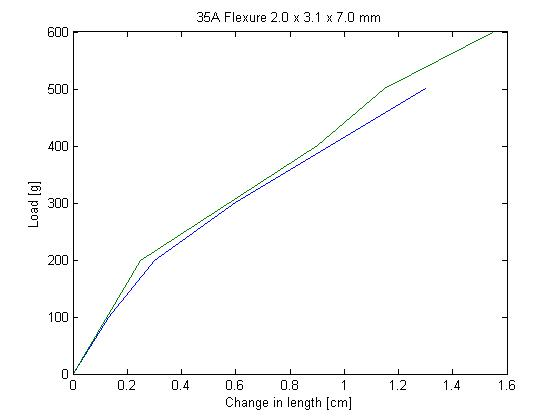## IE-60A:

Actual data:

Length [mm] Change in length [mm] Load [g]
54.01 0 0
60.62 6.61 100
68.5 14.49 200
81.3 27.29 300
97.08 43.07 400
114.08 60.07 500
128.27 74.26 600
139.33 85.32 700
149.02 95.01 800

• 60A Flexture Data: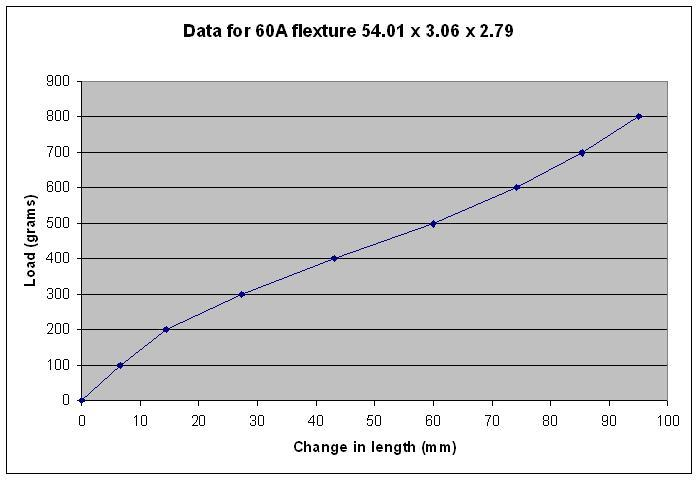## Very old IE-50A:

The flexure measured was the main flexure from a BigToe.
```Weight   F   x   deltax   k=F/dx [N/m]
0g   0N   4.86mm   0mm   --
100g   1N   8.53mm   3.67   272
200g   2N   16.4mm   11.54   173
300g   3N   25.5mm   20.64   145
400g   4N   broke--delaminated after a little while

deltax between 1N and 2N: 16.4-8.53=7.87
k = 1N/7.87mm = 127
==> E = 127*4.86e-3/3.1e-6 = 200,000 = 200kPa

dimensions
1.4mm thick
2.2mm tall (approximately)
==> A ~= 3.1 x 10^-6

E = k*l/A  where l = original length
```

## Vytaflex 20A:

Weight F x deltax k=F/dx [N/m]
0g 0N 2.34mm 0mm --
100g 1N 3.58mm 1.24 806
200g 2N 5.29mm 2.95 678
300g 3N 6.95mm 4.61 651
400g 4N 9.2mm 6.86 583

```using k=650,
E = 650 * 2.34e-3 / 7.83e-6 = 194,000

dimensions:
2.34mm long

1.45mm thick
3 x 0.8mm thick plus 3 x 1.0mm thick

==> Area = 1.45mm x (2.4mm + 3.0mm) = 7.83e-6
```

## Vytaflex 40A:

Length [mm] Change in length [mm] Load [g]
13.5 0 0
15.9 2.4 100
18.7 5.2 200
22.24 8.9 300
28.2 14.7 400
32.1 18.6 500
39 25.5 600
44.5 31 700
52.1 38.6 800
57.4 43.9 900
• 40Aflexturedata.JPG:• 40A: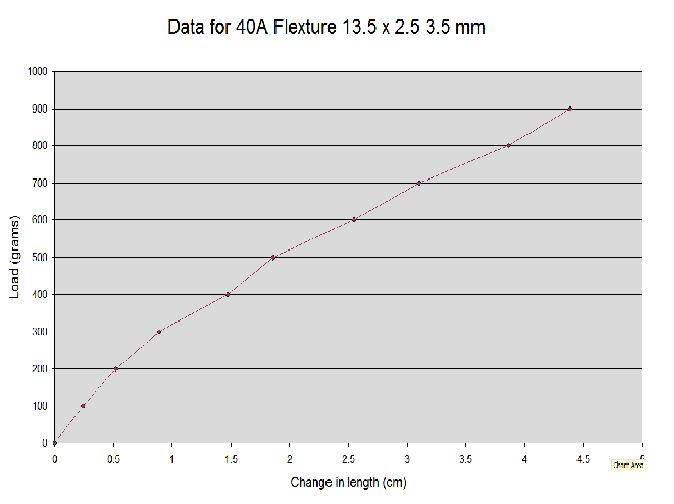Copyright &© by the contributing authors. All material on this collaboration platform is the property of the contributing authors.
Ideas, requests, problems regarding TWiki? Send feedback